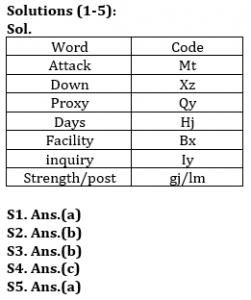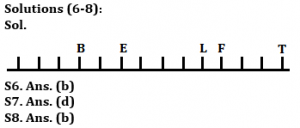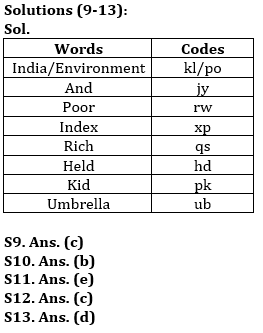Latest Banking jobs   »

# Reasoning Ability Quiz For Bank Foundation 2023 -01st May

Directions (1-5): Study the following information carefully and answer the questions given below:
In a certain code language,
‘Attack proxy down’ is written as ‘xz mt qy’,
‘Proxy days facility’ is written as ‘qy hj bx’,
‘Post strength attack’ is written as ‘lm mt gj’.
‘Down days inquiry’ is written as ‘xz hj iy’,

Q1. What is the code for ‘facility’?
(a) bx
(b) hj
(c) qy
(d) lm
(e) None of these

Q2. Which of the following may be the code for ‘strength down’?
(a) gj hj
(b) lm xz
(c) bx lm
(d) gj iy
(e) None of these

Q3. What is the code for ‘Inquiry’?
(a) hj
(b) iy
(c) xz
(d) lm
(e) Can’t be determined

Q4. What does ‘xz’ stand for?
(a) Post
(b) Strength
(c) Down
(d) days
(e) None of these

Q5. What is the code for ‘Proxy’?
(a) qy
(b) hj
(c) bx
(d) mt
(e)None of these

Direction (6-8): Study the following information carefully and answer the questions given below:
A certain number of persons are sitting in a row facing to the north. B sits 4th from one of the extreme ends of the row. There is one person sits between E and B. There are four persons sit between E and F. T sits 3rd to the right of F. The number of persons sit left to the F is as same as the number of persons sit to the right from B. At least three persons sit between B and F. L sits exactly between E and T but is not an immediate neighbor of E and T.

Q6. How many persons are sitting in the row, as per the given information?
(a) Eight
(b) Fourteen
(c) Ten
(d) Twelve
(e) Can’t be determined

Q7. What is the position of B with respect to the L?
(a) 6th to the right
(b) 5th to the left
(c) 7th to the left
(d) 6th to the left
(e) None of these

Q8. Who among the following person is sitting at the extreme ends of the row?
(a) E
(b) T
(c) F
(d) L
(e) None of these

Direction (9-13): Study the following information carefully and answer the questions given below:
In a certain code language:
“India environment and poor index” is coded as “po rw xp jy kl”
“Rich and Poor held” is coded as “qs jy rw hd”
“Umbrella and rich kid” is coded as “ub jy qs pk”
“Poor index rich umbrella” is coded as “rw xp qs ub”

Q9. What is the code for “held” in the given code language?
(a) qs
(b) rw
(c) hd
(d) jy
(e) None of these

Q10. What is the code for “umbrella index” in the given code language?
(a) xp po
(b) ub xp
(c) rw ub
(d) xp qs
(e) None of these

Q11. What is the code for “India” in the given code language?
(a) jy
(b) po
(c) ub
(d) kl
(e) Either (b) or (d)

Q12. If “Rich and backward” is coded as “qs jy tr” then what may be the possible code for “backward people”?
(a) jy nl
(b) qs tr
(c) tr nl
(d) Either (b) or (c)
(e) None of these

Q13. What is the code for “poor kid index” in the given code language?
(a) rw ub xp
(b) ub jy rw
(c) hd xp jy
(d) rw pk xp
(e) None of these

Q14. Sagar is 20th from the left end of a row and Alok is 12th from the right end of row. If they interchanged their positions, then Alok ranks become 10 from right end. Find total number of persons in the row?
(a) 30
(b) 39
(c) 28
(d) 31
(e) None of these

Q15. Among J, K, L, M and N, each one of them is of different heights, K is taller than only one person. M is shorter than only L. M is taller than J and N (both). Who among them is the third tallest?
(a) J
(b) K
(c) L
(d) N
(e) Either (a) or (d)

SolutionsS14. Ans(e)
Sol. Total number of persons in the row= (20+10-1) =29

S15. Ans.(e)
Sol. L> M > J/N > K > J/N## FAQs

### What is the selection process of the Bank Clerk?

The selection process of the Bank Clerk is Prelims & Mains.

#### Congratulations!Union Budget 2023-24: Free PDF